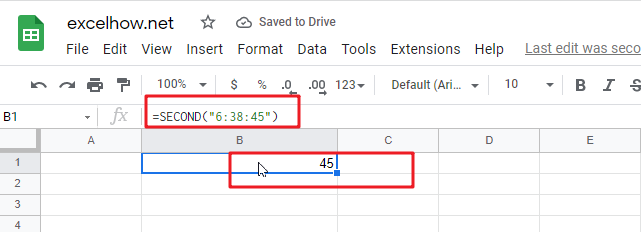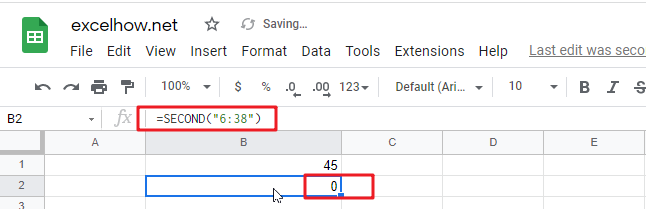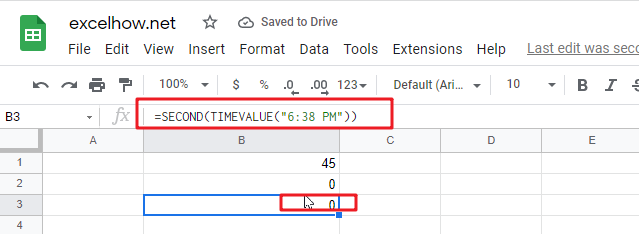# ExcelHow

This post will guide you how to use Google Sheets SECOND function with syntax and examples.

## Description

The Google Sheets SECOND function returns the seconds of a time value. Or returns an integer value that represent the second component of a given excel time. And the return value is between 0-59.

For example, if you pass a time of 6:32:54 PM into SECOND function, and it will return 54. You can use this function to extract the second component from a time value.

The SECOND function can be used to get the second component of a given time in google sheets. The purpose of this function is to get the second as a number from a time and its returned values is a number that representing time. You can use the SECOND function to extract the second into a cell or you can also pass the result into another function as a new formula.

The SECOND function is a build-in function in Google Sheets and it is categorized as a Date function.

## Syntax

The syntax of the SECOND function is as below:

=SECOND (Time )

Where the SECOND function arguments is:

• Time -This is a required argument. The time from which you want to extract the second component. it may be typed as text string within quotation marks (for example, “13:52 PM”), or it can be a decimal numbers (for example, 0.5), or the result of a formula (for example, TIMEVALUE(“13.54:30 PM”)).  It must be a cell reference that containing a date or time, or a function that returning a date/time type or a number.

Note:

• A serial date is how the google sheets stores dates and times and it represents the number of days since 1900-01-01, so the January 1, 1900 date is serial number 1 by default.
• If the `Time` argument is not a valid time, it will return #VALUE! Error.
• `Time `argument can be supplied to the SECOND function as a time text string or as a decimal number. If you wish to create a time value with separate hour, minute and second, you can use the TIME function.

## Google Sheets SECOND Function Examples

The below examples will show you how to use google sheets SECOND Function to return the seconds of a given time value.

1# get the seconds from an Excel time 6:38:45, enter the following formula in Cell B1.

`=SECOND("6:38:45")`2# Get the second portion of the date with no second portion specified, enter the following formula in Cell B3.

`=SECOND("6:38")`or

`=SECOND(TIMEVALUE("6:38 PM"))`It will return 0.